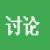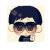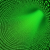## 【c++疑问】如何定义一个[模板类]类型的vector

为什么80%的码农都做不了架构师？->>>hi 大家好：

```template <class T>
class DictItem
{
public:
int insert_elem(T t)
{
_elem_vec.push_back(t);
}
private:
char _item_type;
std::vector<T> _elem_vec;
};```

0
z0
z

```#include <vector>
using namespace std;

struct ItemType
{
int index;
double subIndex;
string name;
};

typedef ItemType T;

class DictItem
{
public:
int insert_elem(T t)
{
_elem_vec.push_back(t);
return 1;
}
private:
char _item_type;
std::vector<T> _elem_vec;
};

int main()
{
DictItem aitem;
return 0;
}```

0```union Item
{
int index;
double subIndex;
string name;
};

void set(Item& item, int& a)
{
item.index = a;
}

void set(Item& item, double& a)
{
item.subIndex = a;
}

void set(Item& item, string& a)
{
item.name = a;
}

template <class T>
class DictItem
{
public:
int insert_elem(T t)
{
Item e;
set(e,t);
_elem_vec.push_back(e);
}

private:
char _item_type;
std::vector<Item> _elem_vec;
};```

0```#include <iostream>
#include <vector>
#include <typeinfo>

using namespace std;

class DictItem{
struct Item{
void *valp;
string _item_type;
}item;
vector<Item> vec;
public:
template<typename T>
void insertItem(const T& val)  // 插入字符串时需显示说明类型，不能用类型推断
{
if(typeid(T) == typeid(char*))
insertItem<string>(string(*(char**)(&val)));
item.valp = (void*)(new T(val));
item._item_type = string(typeid(T).name());
vec.push_back(item);
}

void display() // 测试输出
{
for(auto it:vec) // C++11
{
if(it._item_type == string(typeid(int).name()))
cout << *((int*)it.valp) << endl;
else if(it._item_type == string(typeid(double).name()))
cout << *((double*)it.valp) << endl;
else if(it._item_type == string(typeid(string).name()))
cout << *((string*)it.valp) << endl;
else if(it._item_type == string(typeid(char).name()))
cout << *((char*)it.valp) << endl;
}
}
};

int main()
{
DictItem dictItem;
dictItem.insertItem(35);
dictItem.insertItem(45);
dictItem.insertItem(42.30);
dictItem.insertItem<string>("str");
dictItem.insertItem('r');
dictItem.insertItem<char*>("abcdef");
dictItem.display();
return 0;
}
```